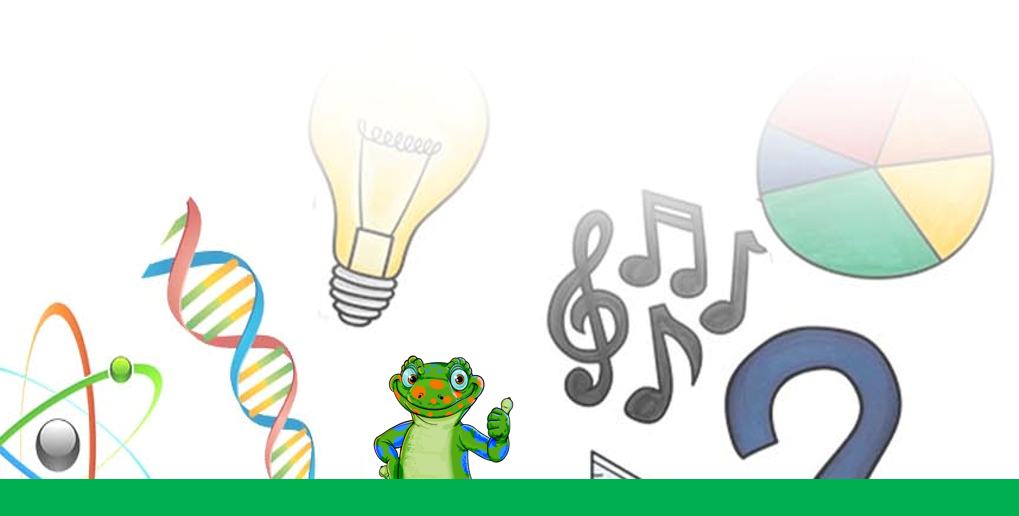### Submit a Resource

Calculus 21 listings

The only thing separating multivariable calculus from ordinary calculus is this newfangled word “multivariable”.

The Precalculus course, often taught in the 12th grade, covers Polynomials; Complex Numbers; Composite Functions; Trigonometric Functions; Vectors; Series, Conic Sections, Probability and Combinatorics.

OpenStax: Pre-Calculus
Openstax

Precalculus is adaptable and designed to fit the needs of a variety of precalculus courses.

Learn AP®︎ Calculus AB for free—everything you need to know about limits, derivatives, and integrals to pass the AP® test.

OpenStax: Calculus Vol. 1
Openstax

Calculus is designed for the typical two- or three-semester general calculus course, incorporating innovative features to enhance student learning.

Learn AP®︎ Calculus BC for free—everything from AP®︎ Calculus AB plus a few extra goodies, such as Taylor series, to prepare you for the AP®︎ test.

OpenStax: Calculus Vol. 2
Openstax

Calculus is designed for the typical two- or three-semester general calculus course, incorporating innovative features to enhance student learning.

Calculus 1. Math:  Fundamental theorem of calculus and definite integrals, Integrals Reverse power rule, Integrals Indefinite integrals of common functions, and more

OpenStax: Calculus Vol. 3
OpenStax

Calculus is designed for the typical two- or three-semester general calculus course, incorporating innovative features to enhance student learning.

Course summary : Integrals review, Integration techniques, Differential equations,  Applications of integrals, Parametric equations, polar coordinates, and more.

youcubed: Polar Graph Art (9-12)
youcubed (Stanford University)

This activity allows students to explore ways to be creative by designing and making a pieces of art with polar graphs.